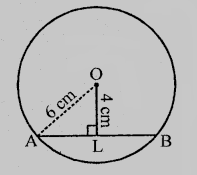# Find the length of a chord which is at a distance of $4\ cm$ from the centre of the circle of radius $6\ cm$.

Given:

A chord is at a distance of $4\ cm$ from the centre of a circle of radius $6\ cm$.

To do:

We have to find the length of the chord.

Solution:Let $AB$ be a chord of a circle with radius $6\ cm$.

$OL \perp AB$

This implies,

$OA = 6\ cm$

$OL = 4\ cm$

$OL$ divides $AB$ into two equal parts.

$AL = LB$

In right angled triangle $OAL$,

$OA^2 = OL^2 + AL^2$                 (Pythagoras Theorem)

$(6)^2 = (4)^2 + AL^2$

$36 = 16 + AL^2$

$AL^2 = 36 - 16 = 20$

$\Rightarrow AL = \sqrt{20}$

$= \sqrt{4\times5}$

$= 2 \times 2.236$

$AB = 2 \times AL = 2 \times 2 \times 2.236$

$= 4 \times 2.236$

$= 8.94\ cm$

The length of the chord is $8.94\ cm$.

Updated on: 10-Oct-2022

89 Views